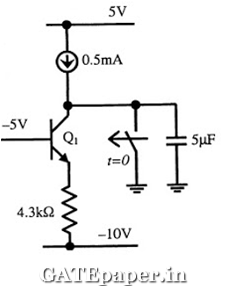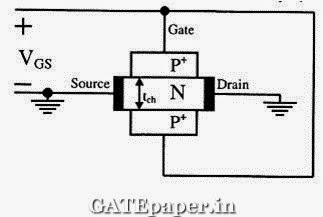### GATE 2011 ECE Video Solutions on EDC (Electronic Devices and Circuits)

1. A silicon PN junction is forward biased with a constant current at room temperature. When the temperature is increased by 10oC, the forward bias voltage across the PN junction
a. Increases by 60 mV
b. Decreases by 60 mV
c. Increases by 25 mV
d. Decreases by 25 mV

2. Drift current in semiconductors depends upon
a. Only the electric field
b. Only the carrier concentration gradient
c. Both the electric field and the carrier concentration
d. Both the electric field and the carrier concentration gradient

3. A Zener diode when used in voltage stabilization circuits, is biased in
a. Reverse bias region below the breakdown voltage
b. Reverse breakdown region
c. Forward bias region
d. Forward bias constant current mode

4. For the BJT Q1 in the circuit shown, β = ∞, VBEon = 0.7 volts, VCEsat = 0.7 volts. The switch is initially closed. At time t=0, the switch is opened. The time at which Q1 leaves the active region isa. 10 ms
b. 25 ms
c. 50 ms
d. 100 ms

5. In the circuit shown below, for the MOS transistors, µncox = 100 µA/V2 and the threshold voltage VT = 1 volt. The voltage VX at the source of the upper transistor isa. 1 volt
b. 2 volts
c. 3 volts
d. 0.367 volts

6. For a BJT, the common base current gain α = 0.98 and the collector base junction reverse bias saturation current, ICO = 0.6 µA. This BJT is connected in the common emitter mode and operated in the active region with a base current (IB) of 20 µA. The collector current IC for this mode of operation is
a. 0.98 mA
b. 0.99 mA
c. 1.0 mA
d. 1.01 mA

7. Common Data Question:
The channel resistance of an N channel JFET is shown below is 600 Ω, when the full channel thickness (tOX) of 10 µm is available for conduction. The built in voltage of the gate P+N junction is (Vbi) is -1 volts. When the gate to source voltage (VGS) is 0 volts, the channel is depleted by 1 µm on each side due to the built in voltage and hence the thickness available for conduction is only 8 µm.i. The channel resistance when VGS = 0 volts is
a. 480 Ω
b. 600 Ω
c. 750 Ω
d. 1000 Ω

ii. The channel resistance when VGS = -3 volt is
a. 360 Ω
b. 917 Ω
c. 1000 Ω
d. 3000 Ω